# Ice Ih

Ice Ih (hexagonal ice) is a proton disordered ice phase having the space group P63/mmc. Ice Ih has the following lattice parameters at 250 K: a=4.51842 Å,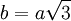$b=a\sqrt3$, and c=7.35556 Å with four molecules per unit cell (in Table 3 of ). The proton ordered form of ice Ih is known as ice XI, which (in principle) forms when ice Ih is cooled to below 72K (it is usually doped with KOH to aid the transition).

## Melting point

The following is a collection of melting points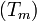$(T_m)$ for the ice Ih-water transition (experimental value is 273.15 K at 1 bar):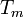$T_m$ Pressure Water model/technique Reference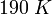$190~K$ 1 bar SPC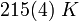$215(4)~K$ 1 bar SPC/E / free energy calculation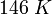$146~K$ 1 bar TIP3P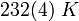$232(4)~K$ 1 bar TIP4P / free energy calculation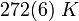$272(6)~K$ 1 bar TIP4P/Ice / free energy calculation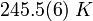$245.5(6)~K$ 1 bar TIP4P/Ew / free energy calculation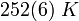$252(6)~K$ 1 bar TIP4P/2005 / free energy calculation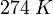$274~K$ 1 bar TIP5P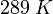$289~K$ 1 bar NvdE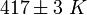$417\pm 3~K$ 2500 bar Perdew-Burke-Ernzerhof functional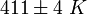$411 \pm 4~K$ 10,000 bar Becke-Lee-Yang-Parr functional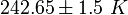$242.65 \pm 1.5~K$ 1 bar TTM2.1-F (classical)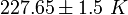$227.65 \pm 1.5~K$ 1 bar TTM2.1-F (quantum)